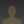# How to Connect Water Flow Sensor with Arduinoakanzler007 wrote 06/30/2020 at 09:51 • 5 min read • Like

Water management is very important in today’s time to tackle the water crises. Supplying water according to the real requirement is important and thus measuring water is a very essential step in water management systems.  There are many water flow measurement techniques as well as different types of water flow meters used to measure the volume of water flow in pipelines but these all are too costly. Through this guide you will get an ideas for design and development of low-cost with the help of readily-available and low-cost water flow sensors.

YF-S201 Water Flow Sensor

Water Flow Sensor, as the name suggests, is a device to measure the flow of water. The Water Flow Sensor used in this project is shown in the image below.

Accurate flow measurement is an essential step both in the terms of qualitative and economic points of view. Flow meters have proven excellent devices for measuring water flow, and now it is very easy to build a water management system using the renowned water flow sensor YF-S201. This sensor sits in line with the water line and contains a pinwheel sensor to measure how much water has moved through it. There is an integrated magnetic Hall-Effect sensor that outputs an electrical pulse with every revolution.

Working of the Water Sensor

The sensor has 3 wires RED, YELLOW, and BLACK as shown in the figure below. The red wire is used for supply voltage which ranges from 5V to 18V and the black wire is connected to GND. The yellow wire is used for output (pulses), which can be read by an MCU. The water flow sensor consists of a pinwheel sensor that measures the quantity of liquid that has passed through it.

Basically, the YF-S201 Water Flow Sensor consists of a Flap Wheel (or a Turbine Wheel) that spins when water flows through the sensor. At the centre of this flap wheel, there is magnet fixed. Keeping this in mind, when the water flows through the YF-S201 Water Flow Sensor, the flap wheel spins due to the force of the water and as a result, the magnet attached to it will also spin. As a result, the magnetic field near the Hall-effect sensor switches polarity as the flap wheel spins and the output of the sensor (on the output Pin – Yellow Wire) will be a pulse.

By keeping track of the number of pulses from the output of the Water Flow Sensor, you can easily calculate the amount of water flowing through the sensor and as a result the Water Flow Rate.

Connections

• Connect the Red and Black wires of the YF-S201 Water Flow Sensor to +5V and GND.
• Since will be used the Interrupt feature of the Arduino, only Digital I/O Pins 2 and 3 are possible to connect to the Output of the Water Flow Sensor.

Code

The code for the Arduino Water Flow Sensor Interface is given below.

```volatile int flow_frequency; // Measures flow sensor pulses
unsigned int l_hour; // Calculated litres/hour
unsigned char flowsensor = 2; // Sensor Input
unsigned long currentTime;
unsigned long cloopTime;
void flow () // Interrupt function
{   flow_frequency++;
}
void setup()
{   pinMode(flowsensor, INPUT);
digitalWrite(flowsensor, HIGH); // Optional Internal Pull-Up
Serial.begin(9600);
attachInterrupt(0, flow, RISING); // Setup Interrupt
sei(); // Enable interrupts
currentTime = millis();
cloopTime = currentTime;
}
void loop ()
{   currentTime = millis();
// Every second, calculate and print litres/hour
if(currentTime >= (cloopTime + 1000))
{
cloopTime = currentTime; // Updates cloopTime
// Pulse frequency (Hz) = 7.5Q, Q is flow rate in L/min.
l_hour = (flow_frequency * 60 / 7.5); // (Pulse frequency x 60 min) / 7.5Q = flowrate in L/hour
flow_frequency = 0; // Reset Counter
Serial.print(l_hour, DEC); // Print litres/hour
Serial.println(" L/hour");   }
}```

The output of the project is to display the quantity of water flowing through the sensor in litres per hour as shown below.

Since the output of the sensor is a pulse, by calculating the frequency of the pulse, we can calculate the volume of the water flowing through the sensor.

The pulse frequency in Hz is 7.5 * Flow Rate (in Litres per minute). So, the quantity of water in Litres per Hour is Pulse Frequency * 60 / 7.5.

Thus now you can calculate the rate of flow of water with the help of this water sensor. See More of Water sensors and try building your water sensing unit.

This guide was written in reference to the tutorial published by electronicshub.com

## Discussions